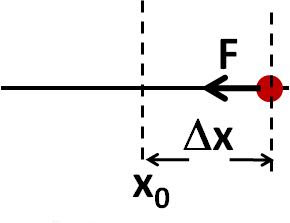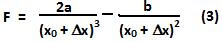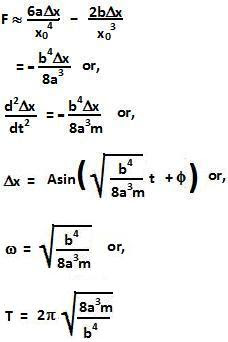## Friday, June 11, 2010

### Irodov Problem 4.17Similar to Problem 4.16,At the equilibrium point xo, F=0, hence we have,Now let us consider the motion of the particle due to a small displacementabout xo. The force will be given by,Using Taylor's series we can approximate (3) as,1.i didn't understood the taylor's series

2.i got the solution of this problem by equating w*w*x=acceleration so obtained and by doing some approximations. but answer is not matching with the answer given in book.

3.i also obtained the same answer as you obtained. But according to the edition of my irodov there is a difference of factor of square root of 2. I request you to confirm the answer

4.Answer did not match with the 1 in my book

5.6.Answer did not match with my book.

7.8.